# Colour Matching Functions¶

The colour matching functions are the tristimulus values of monochromatic stimuli of equal radiant power  and are at the hearth of the CIE Colour System, their creation history has been briefly introduced in the Spectrum notebook.

Colour provides the following spectral sensitivity and colour matching functions:

In :
import colour

sorted(colour.colorimetry.LMS_CMFS.keys())

Out:
['Smith & Pokorny 1975 Normal Trichromats',
'Stockman & Sharpe 10 Degree Cone Fundamentals',
'Stockman & Sharpe 2 Degree Cone Fundamentals']
In :
sorted(colour.colorimetry.RGB_CMFS.keys())

Out:
['Stiles & Burch 1955 2 Degree RGB CMFs',
'Stiles & Burch 1959 10 Degree RGB CMFs',
'Wright & Guild 1931 2 Degree RGB CMFs']
In :
sorted(colour.colorimetry.STANDARD_OBSERVERS_CMFS.keys())

Out:
['CIE 1931 2 Degree Standard Observer',
'CIE 1964 10 Degree Standard Observer',
'CIE 2012 10 Degree Standard Observer',
'CIE 2012 2 Degree Standard Observer',
'cie_10_1964',
'cie_2_1931']

Note: 'cie_2_1931' and 'cie_10_1964' are convenient aliases for respectively 'CIE 1931 2 Degree Standard Observer' and 'CIE 1964 10 Degree Standard Observer'.

In :
sorted(colour.CMFS.keys())

Out:
['CIE 1931 2 Degree Standard Observer',
'CIE 1964 10 Degree Standard Observer',
'CIE 2012 10 Degree Standard Observer',
'CIE 2012 2 Degree Standard Observer',
'Smith & Pokorny 1975 Normal Trichromats',
'Stiles & Burch 1955 2 Degree RGB CMFs',
'Stiles & Burch 1959 10 Degree RGB CMFs',
'Stockman & Sharpe 10 Degree Cone Fundamentals',
'Stockman & Sharpe 2 Degree Cone Fundamentals',
'Wright & Guild 1931 2 Degree RGB CMFs',
'cie_10_1964',
'cie_2_1931']

Most of them are sourced from the Colour & Vision Research Laboratory database.

## Tristismulus Space¶

Colour stimuli can be represented by vectors into a three-dimensionl space called tristimulus space. They are usually denoted by boldface letters such as $\textbf{Q}$, $\textbf{R}$, $\textbf{G}$ and $\textbf{B}$ where $\textbf{Q}$ represents a test colour stimulus and $\textbf{R}$, $\textbf{G}$ and $\textbf{B}$ are reserved for the fixed primary stimuli chosen for the colour matching experiment. 

A colour match between an test colour stimulus $\textbf{Q}$ and the additive mixture of the three fixed primary stimuli $\textbf{R}$, $\textbf{G}$ and $\textbf{B}$ is expressed by the following equation: 

$$\begin{equation} \textbf{Q}=R_Q\textbf{R}+G_Q\textbf{G}+B_Q\textbf{B} \end{equation}$$

where the multipliers $R_Q$,$G_Q$,$B_Q$ measured in terms of the assigned respective units of the $\textbf{R}$, $\textbf{G}$ and $\textbf{B}$ primary stimuli are called the tristimulus values of $\textbf{Q}$.

A monochromatic stimulus $\textbf{Q}_\lambda$ of wavelength $\lambda$ is the quantity $\lbrace P_\lambda d\lambda\rbrace$ that represents the radiant power in the wavelength interval of width $d\lambda$ centered at the wavelength $\lambda$ and can be expressed by the following equation: 

$$\begin{equation} \textbf{Q}_\lambda=R_\lambda\textbf{R}+G_\lambda\textbf{G}+B_\lambda\textbf{B} \end{equation}$$

where $R_\lambda$,$G_\lambda$,$B_\lambda$ are called the spectral tristimulus values of $\textbf{Q}_\lambda$.

The equation for a colour match involving a monochromatic constituent $\textbf{E}_\lambda$ of the equal energy stimulus $\textbf{E}$ is:

$$\begin{equation} \textbf{E}_\lambda=\bar{r}(\lambda)\textbf{R}+\bar{g}(\lambda)\textbf{G}+\bar{b}(\lambda)\textbf{B} \end{equation}$$

where $\bar{r}(\lambda)$,$\bar{g}(\lambda)$,$\bar{b}(\lambda)$ are called the spectral tristimulus values of $\textbf{E}_\lambda$. The lowercase notation with an overbar indictates a special set of tristimulus values and is the standard notation for colour matching functions.

In :
from colour.plotting import *

In :
colour_style();

In :
# Plotting the spectral distribution of a colour stimulus *E* having an
# equal energy spectrum.
sd = colour.SpectralDistribution(
dict((i, 1.) for i in range(360, 830)), name='E')
plot_single_sd(sd, bounding_box=[360, 830, 0, 1.5]);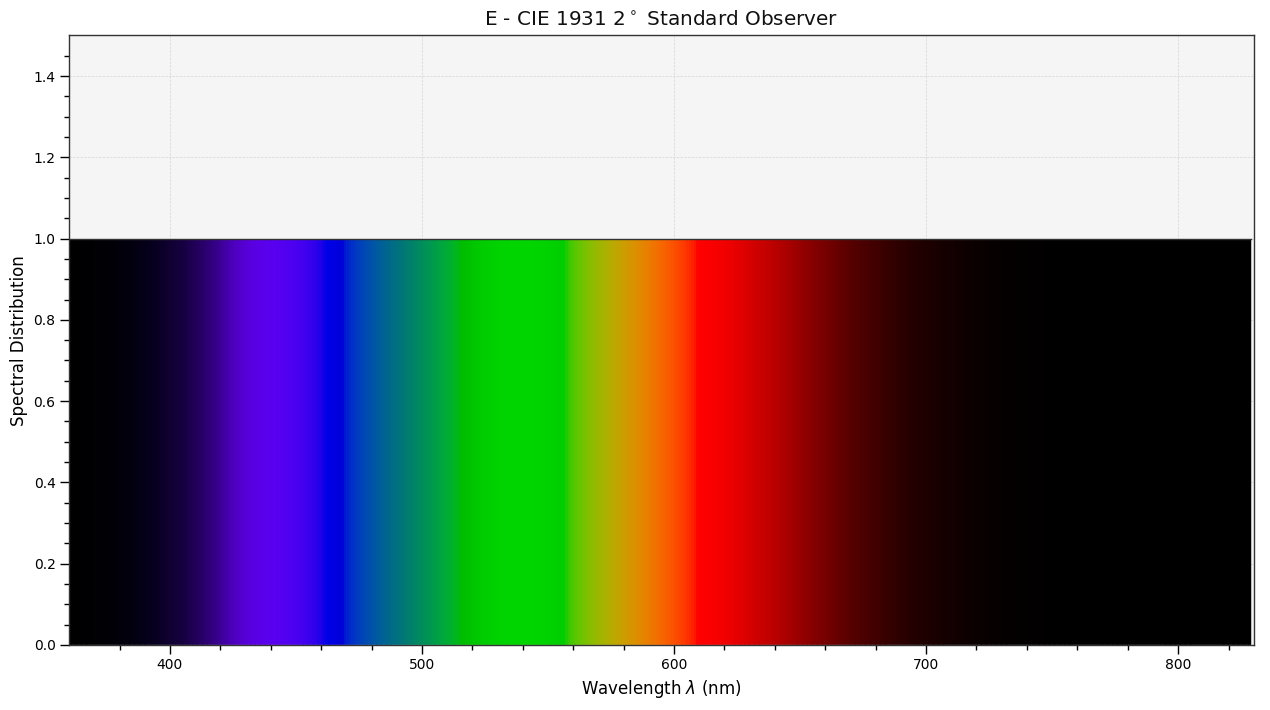## $\bar{r}(\lambda)$,$\bar{g}(\lambda)$,$\bar{b}(\lambda)$ Colour Matching Functions¶

### Wright & Guild 1931 2° RGB CMFs¶

Wright & Guild 1931 2° RGB CMFs $\bar{r}(\lambda)$,$\bar{g}(\lambda)$,$\bar{b}(\lambda)$ colour matching functions are derived from the two independent experiments of Wright (1928) and Guild (1931). 

Both determined the chromaticity coordinates of a set of monochromatic stimuli in the wavelength range 400-700 nm using a circular 2° angular subtense bipartite field whose image is centered at the fovea of retina to avoid any participation of rod vision in the measurements.

The bottom half of the field was illuminated by the test colour stimulus to be matched and the top half was illuminated by the fixed primary stimuli at respective fixed wavelengths of 700 nm, 546.1 nm and 435.8 nm but adjustable intensity.

The observer would adjust the intensity of each of the three fixed primary stimuli until both halves of the field were colour matched.

Not all the test colour stimuli could be matched using this technique. For example, matching a yellow test colour stimulus required large amounts of red and green fixed primary stimuli. If the resulting fixed primary stimuli mixture was less yellow than the test colour stimulus, a variable amount of blue fixed primary stimulus could be added to the the test colour stimulus. For these cases, the amount of fixed primary stimulus added to the test colour stimulus was considered as a negative value.

For example, the test colour stimulus $\textbf{E}_\lambda$ at $\lambda=475$ and fixed primary stimuli values read off at $\bar{r}(475)=-0.045$, $\bar{g}(475)=0.032$ and $\bar{b}(475)=0.186$ can be expressed with the following equation: 

$$\begin{equation} \textbf{E}_{475}=-0.045\textbf{R}+0.032\textbf{G}+0.186\textbf{B} \end{equation}$$

The chromaticity coordinates $r(\lambda)$,$g(\lambda)$,$b(\lambda)$ of the monochromatic stimuli can then be calculated:

$$\begin{equation} r(\lambda)=\cfrac{\bar{r}(\lambda)}{\bar{r}(\lambda)+\bar{g}(\lambda)+\bar{b}(\lambda)}\\ g(\lambda)=\cfrac{\bar{g}(\lambda)}{\bar{r}(\lambda)+\bar{g}(\lambda)+\bar{b}(\lambda)}\\ b(\lambda)=\cfrac{\bar{b}(\lambda)}{\bar{r}(\lambda)+\bar{g}(\lambda)+\bar{b}(\lambda)} \end{equation}$$

with $$\begin{equation} r(\lambda)+g(\lambda)+b(\lambda)=1 \end{equation}$$

Wright & Guild 1931 2° RGB CMFs $\bar{r}(\lambda)$,$\bar{g}(\lambda)$,$\bar{b}(\lambda)$ colour matching functions have the following range and increments:

In :
colour.colorimetry.RGB_CMFS['Wright & Guild 1931 2 Degree RGB CMFs'].shape

Out:
SpectralShape(380.0, 780.0, 5.0)
In :
plot_single_cmfs('Wright & Guild 1931 2 Degree RGB CMFs',
legend_location='lower right');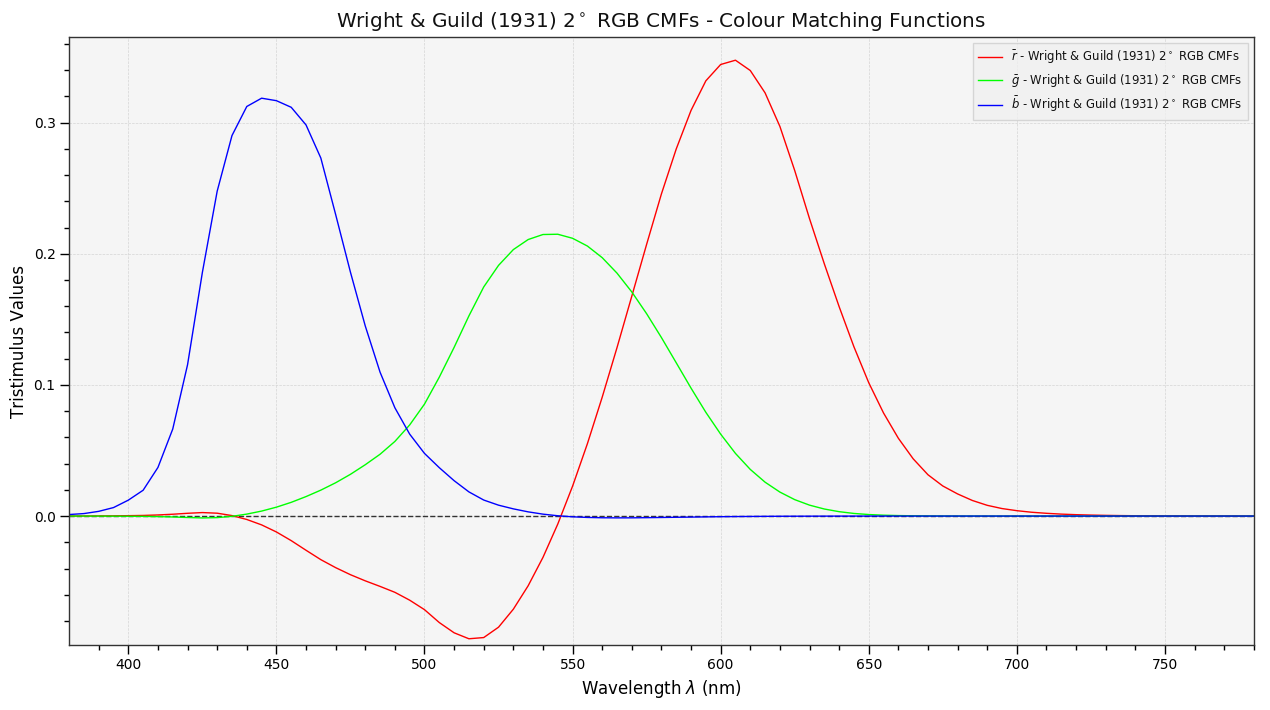### Stiles & Burch 1955 2° RGB CMFs¶

Stiles & Burch 1955 2° RGB CMFs $\bar{r}(\lambda)$,$\bar{g}(\lambda)$,$\bar{b}(\lambda)$ colour matching functions are the result of a major re-examination of the CIE standard by Stiles in the 1950's because of concern about the correctness of the CIE 1931 2° Standard Observer data regarding: 

• The luminous efficiency function $V(\lambda)\equiv\bar{y}(\lambda)$ in the visible spectrum ends, especially the blue end.
• The inconsistency between computed and observed colour computation differences of certain titanium pigments.
• The suitability of doing colour matching in large visual fields as used in industrial colour production control.

In 1955, the CIE Colorimetry Comittee decided that the discrepancies between the CIE 1931 2° Standard Observer and the new data from the interim report by Stiles (1955), though significant from an experimental aspect were not large enough to modify the standard for practical colorimetry.

Stiles & Burch 1955 2° RGB CMFs $\bar{r}(\lambda)$,$\bar{g}(\lambda)$,$\bar{b}(\lambda)$ colour matching functions have the following range and increments:

In :
colour.colorimetry.RGB_CMFS['Stiles & Burch 1955 2 Degree RGB CMFs'].shape

Out:
SpectralShape(390.0, 730.0, 5.0)
In :
plot_single_cmfs('Stiles & Burch 1955 2 Degree RGB CMFs',
legend_location='upper left');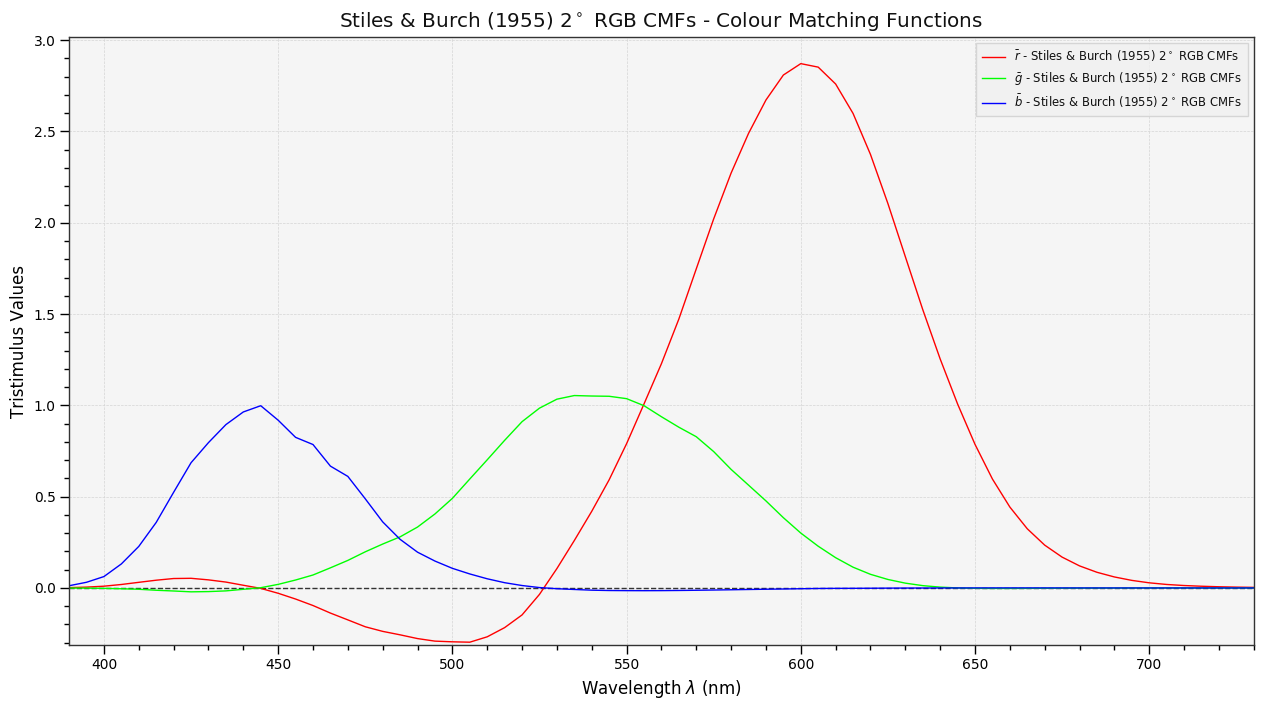### Stiles & Burch 1959 10° RGB CMFs¶

Stiles following the direction recommenced by CIE Colorimetry Committee continued his work and focused on large-field colour matching experiments.

In 1958 the final report of the National Physical Laboratory (N.P.L) colour-matching investigation by Stiles and Burch (1959) proposed the Stiles & Burch 1959 10° RGB CMFs $\bar{x}_{10}(\lambda)$,$\bar{y}_{10}(\lambda)$,$\bar{z}_{10}(\lambda)$ colour matching functions. 

Stiles & Burch 1959 10° RGB CMFs $\bar{x}_{10}(\lambda)$,$\bar{y}_{10}(\lambda)$,$\bar{z}_{10}(\lambda)$ colour matching functions have the following range and increments:

In :
colour.colorimetry.RGB_CMFS['Stiles & Burch 1959 10 Degree RGB CMFs'].shape

Out:
SpectralShape(390.0, 830.0, 5.0)
In :
plot_single_cmfs('Stiles & Burch 1959 10 Degree RGB CMFs',
legend_location='upper left');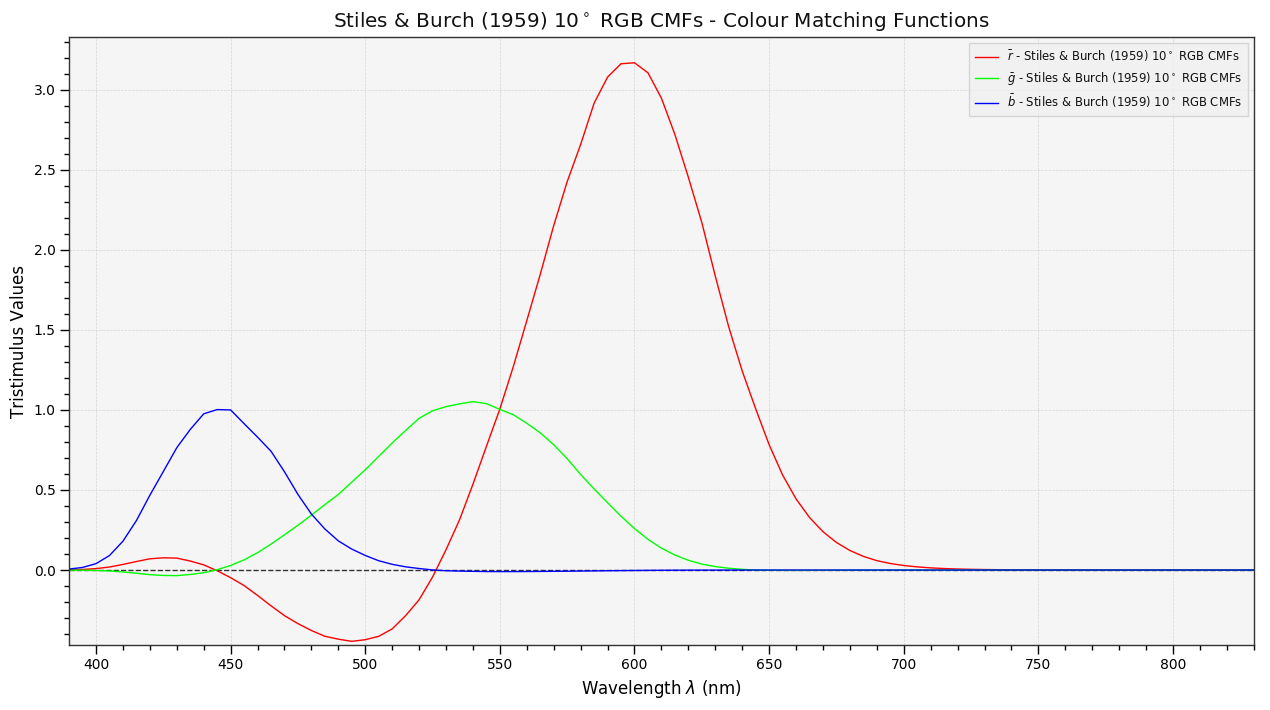In :
# Comparing *Stiles & Burch 1955 2 Degree RGB CMFs* and
# *Stiles & Burch 1959 10 Degree RGB CMFs* colour matching functions.
plot_multi_cmfs(['Stiles & Burch 1955 2 Degree RGB CMFs',
'Stiles & Burch 1959 10 Degree RGB CMFs']);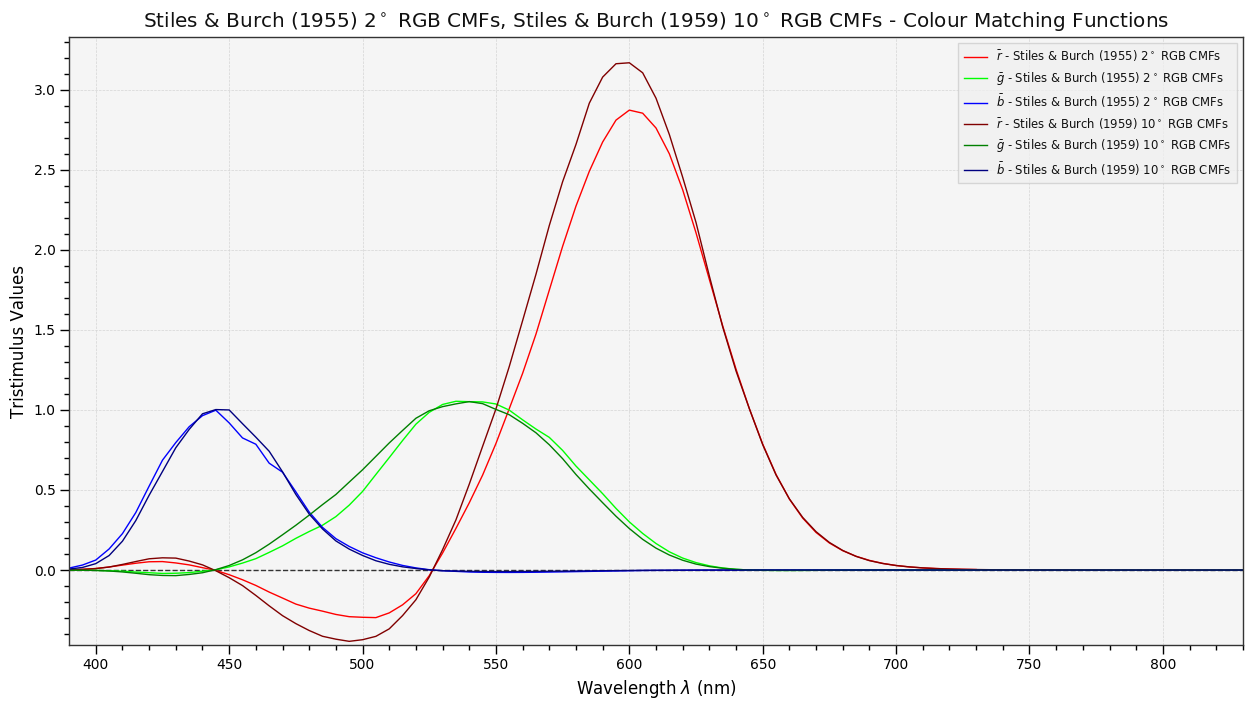## $\bar{x}(\lambda)$ $\bar{y}(\lambda)$ $\bar{z}(\lambda)$ Colour Matching Functions¶

### CIE 1931 2° Standard Observer¶

Wright & Guild 1931 2° RGB CMFs $\bar{r}(\lambda)$,$\bar{g}(\lambda)$,$\bar{b}(\lambda)$ colour matching functions have negative values that are inconvenient for various reasons:

• Computation of the $\textbf{R}$,$\textbf{G}$,$\textbf{B}$ tristimulus values of a given stimulus $\textbf{Q}$ defined by a spectral distribution $\lbrace P_\lambda d\lambda\rbrace$ are done according to the following equations: 

$$\textbf{R}=\int_{\lambda_a}^{\lambda_b}P_\lambda\bar{r}(\lambda)d\lambda\\ \textbf{G}=\int_{\lambda_a}^{\lambda_b}P_\lambda\bar{g}(\lambda)d\lambda\\ \textbf{B}=\int_{\lambda_a}^{\lambda_b}P_\lambda\bar{b}(\lambda)d\lambda$$

This computation involves summing separately products with positive and negative signs and then a final differencing of the sums.

• Computation of photometric quantities like luminance for a given stimulus requires either another direct integration using the luminous efficiency $V(\lambda)$ function or the evaluation of a linear combination of the previously computed tristimulus values.
• Development of direct-reading colorimeters is more difficult because of the sign change.

Those reasons lead the CIE to transform the Wright & Guild 1931 2° RGB CMFs $\bar{r}(\lambda)$,$\bar{g}(\lambda)$,$\bar{b}(\lambda)$ colour matching functions into a new set of functions based on new primary stimuli $X$, $Y$, $Z$: The CIE 1931 2° Standard Observer $\bar{x}(\lambda)$,$\bar{y}(\lambda)$,$\bar{z}(\lambda)$ colour matching functions.

Transformation from CIE RGB colourspace $r$,$g$,$b$ chromaticity coordinates to CIE XYZ colourspace $x$,$y$,$z$ chromaticity coordinates is expressed as follows: 

$$\begin{equation} x=\cfrac{0.49000r+0.31000g+0.20000b}{0.66697r+1.13240g+1.20063b}\\ y=\cfrac{0.17697r+0.81240g+0.01063b}{0.66697r+1.13240g+1.20063b}\\ z=\cfrac{0.00000r+0.01000g+0.99000b}{0.66697r+1.13240g+1.20063b} \end{equation}$$

Then the $X$, $Y$ and $Z$ tristimulus values are calculated as follows: 

$$\begin{equation} X=\cfrac{x}{y}V,\qquad Y=V,\qquad Z=\cfrac{z}{y}V \end{equation}$$

where $V$ is the luminance of the stimulus calculated in accordance with the luminous efficiency function $V(\lambda)$.

Transformation of the monochromatic stimuli chromaticity coordinates $r(\lambda)$,$g(\lambda)$,$b(\lambda)$ into the $\bar{x}(\lambda)$,$\bar{y}(\lambda)$,$\bar{z}(\lambda)$ colour matching functions also uses the above equation: 

$$\begin{equation} \bar{x}(\lambda)=\cfrac{x(\lambda)}{y(\lambda)}V(\lambda),\qquad \bar{y}(\lambda)=V(\lambda),\qquad \bar{z}(\lambda)=\cfrac{z(\lambda)}{y(\lambda)}V(\lambda) \end{equation}$$

The colour.RGB_2_degree_cmfs_to_XYZ_2_degree_cmfs definition is provided for educational purpose to illustrate the transformation from Wright & Guild 1931 2° RGB CMFs $\bar{r}(\lambda)$,$\bar{g}(\lambda)$,$\bar{b}(\lambda)$ colour matching functions into the CIE 1931 2° Standard Observer $\bar{x}(\lambda)$,$\bar{y}(\lambda)$,$\bar{z}(\lambda)$ colour matching functions:

In :
print(colour.colorimetry.RGB_2_degree_cmfs_to_XYZ_2_degree_cmfs(435))
print(colour.colorimetry.STANDARD_OBSERVERS_CMFS['CIE 1931 2 Degree Standard Observer'])

[ 0.32884511  0.01684     1.62465501]
[ 0.3285   0.01684  1.62296]


The CIE 1931 2° Standard Observer $\bar{x}(\lambda)$,$\bar{y}(\lambda)$,$\bar{z}(\lambda)$ colour matching functions have the following range and increments:

In :
colour.colorimetry.STANDARD_OBSERVERS_CMFS['CIE 1931 2 Degree Standard Observer'].shape

Out:
SpectralShape(360.0, 830.0, 1.0)
In :
plot_single_cmfs('CIE 1931 2 Degree Standard Observer');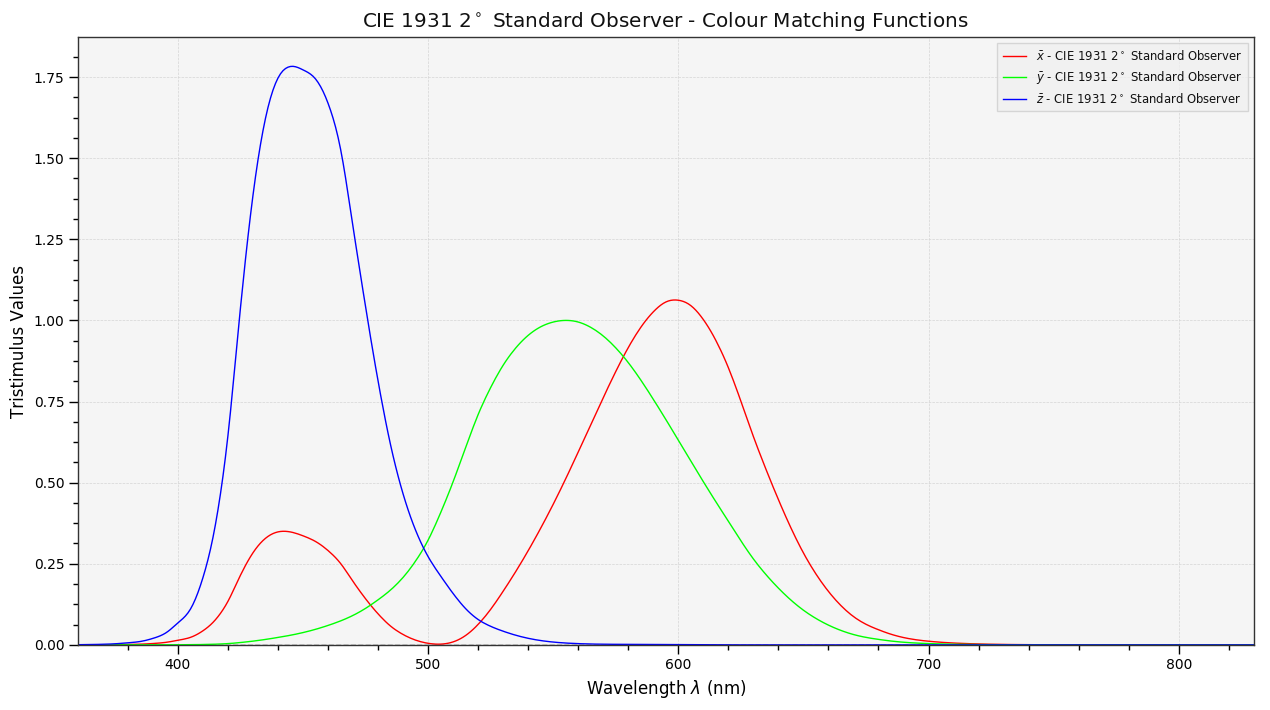### CIE 1964 10° Standard Observer¶

The CIE 1964 10° Standard Observer $\bar{x}_{10}(\lambda)$,$\bar{y}_{10}(\lambda)$,$\bar{z}_{10}(\lambda)$ colour matching functions are the result of Stiles and Burch (1959) and Speranskaya (1959) investigations with large-field colour matching experiments.

Judd, acting for the CIE averaged, Stiles and Burch (1959) and Speranskaya (1959) colour matching functions after correcting Speranskaya data for rod intrusion (CIE, 1959).

Building upon Judd new set of data, the CIE following the same general model than for the CIE 1931 2° Standard Observer created a new coordinate system. The equations to convert from the $\bar{r}_{10}(\lambda)$,$\bar{g}_{10}(\lambda)$,$\bar{b}_{10}(\lambda)$ colour matching functions to the new $\bar{x}_{10}(\lambda)$,$\bar{y}_{10}(\lambda)$,$\bar{z}_{10}(\lambda)$ colour matching functions are as follows: 

$$\begin{equation} \bar{x}_{10}(\lambda)=0.341080\bar{r}_{10}(\lambda)+0.189145\bar{g}_{10}(\lambda)+0.387529\bar{b}_{10}(\lambda)\\ \bar{y}_{10}(\lambda)=0.139058\bar{r}_{10}(\lambda)+0.837460\bar{g}_{10}(\lambda)+0.073316\bar{b}_{10}(\lambda)\\ \bar{z}_{10}(\lambda)=0.000000\bar{r}_{10}(\lambda)+0.039553\bar{g}_{10}(\lambda)+2.026200\bar{b}_{10}(\lambda) \end{equation}$$

The colour.RGB_10_degree_cmfs_to_XYZ_10_degree_cmfs definition is provided for educational purpose to illustrate the transformation from Stiles & Burch 1959 10° RGB CMFs $\bar{x}_{10}(\lambda)$,$\bar{y}_{10}(\lambda)$,$\bar{z}_{10}(\lambda)$ colour matching functions into the CIE 1931 2° Standard Observer $\bar{r}_{10}(\lambda)$,$\bar{g}_{10}(\lambda)$,$\bar{b}_{10}(\lambda)$ colour matching functions:

In :
print(colour.colorimetry.RGB_10_degree_cmfs_to_XYZ_10_degree_cmfs(435))
print(colour.colorimetry.STANDARD_OBSERVERS_CMFS['CIE 1964 10 Degree Standard Observer'])

[ 0.35408714  0.04904404  1.7775067 ]
[ 0.357719  0.049602  1.7985  ]


The CIE 1964 10° Standard Observer $\bar{x}_{10}(\lambda)$,$\bar{y}_{10}(\lambda)$,$\bar{z}_{10}(\lambda)$ colour matching functions have the following range and increments:

In :
colour.colorimetry.STANDARD_OBSERVERS_CMFS['CIE 1964 10 Degree Standard Observer'].shape

Out:
SpectralShape(360.0, 830.0, 1.0)
In :
plot_single_cmfs('CIE 1964 10 Degree Standard Observer');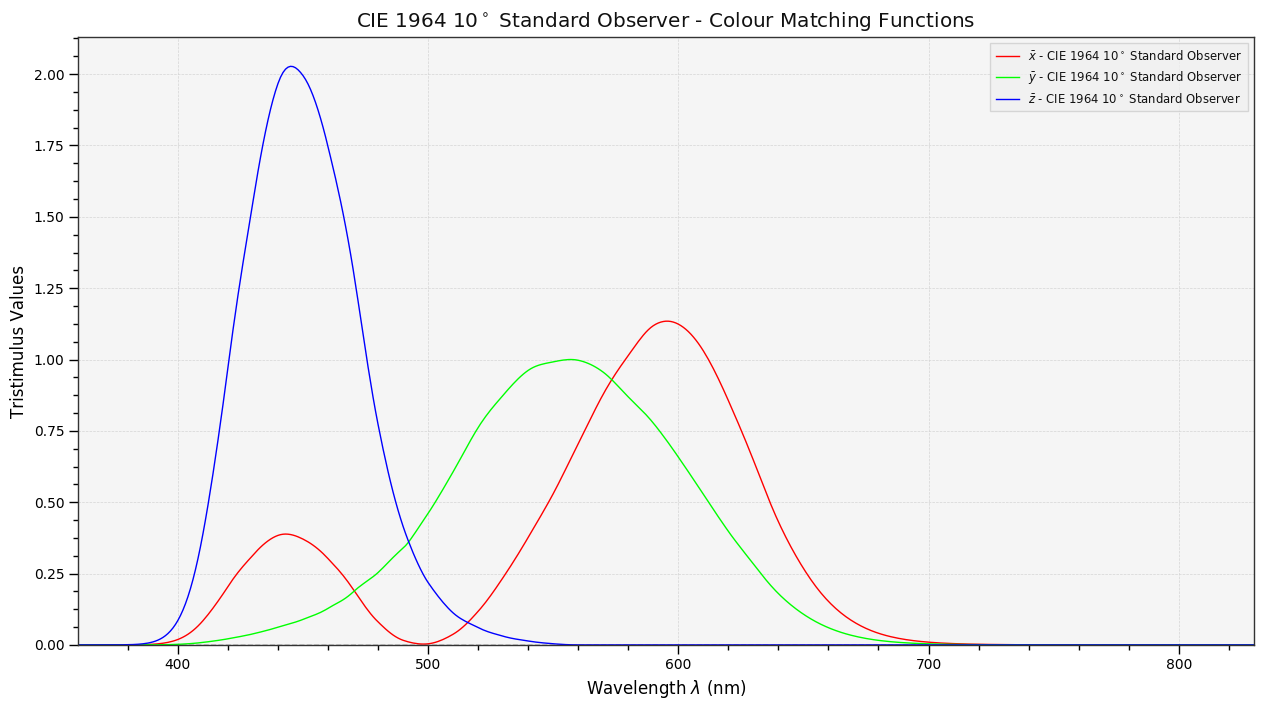In :
# Comparing *CIE 1931 2 Degree Standard Observer* and
# *CIE 1964 10 Degree Standard Observer* colour matching functions.
plot_multi_cmfs(['CIE 1931 2 Degree Standard Observer',
'CIE 1964 10 Degree Standard Observer']);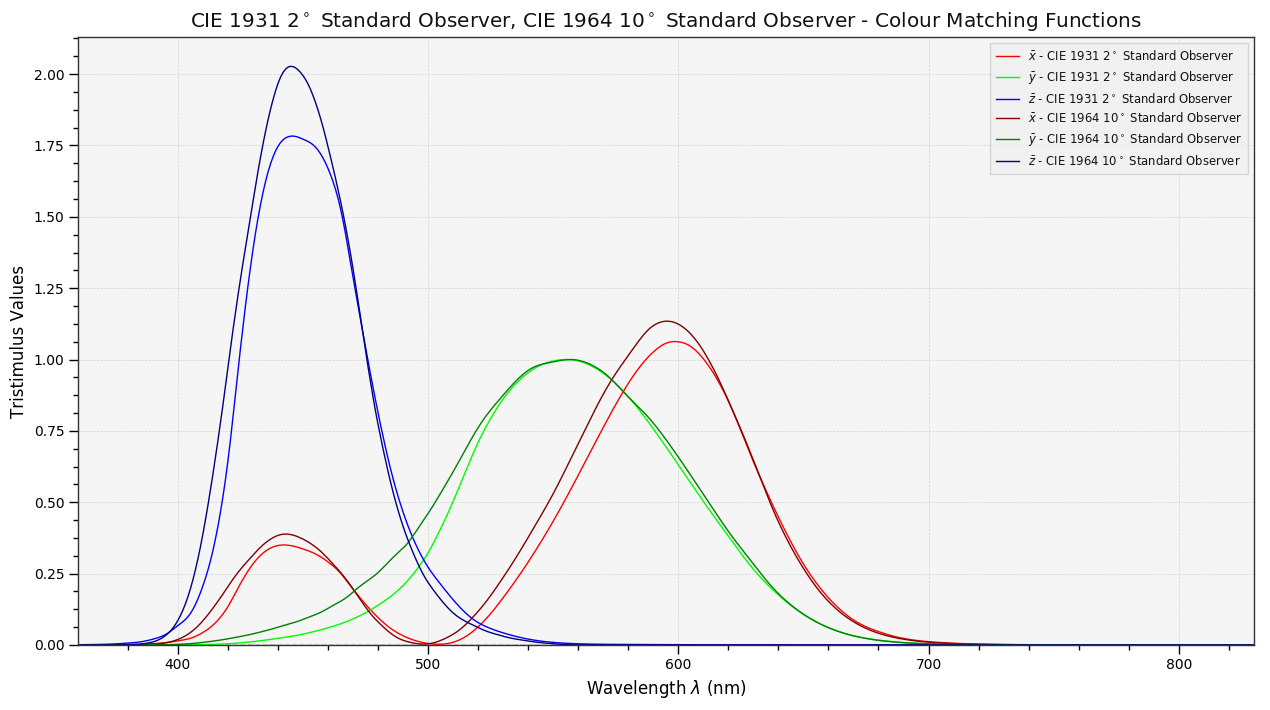### CIE 2012 2° Standard Observer¶

The CIE 2012 2° Standard Observer $\bar{r}(\lambda)$,$\bar{g}(\lambda)$,$\bar{b}(\lambda)$ colour matching functions are linear transformations of Stockman & Sharpe 2° Cone Fundamentals $\bar{l}(\lambda)$,$\bar{m}(\lambda)$,$\bar{s}(\lambda)$ colour matching functions.

They are recommended by the CIE TC 1-36 technical commitee report (CIE 170-1:2006, 2006) as the new physiologically relevant fundamental CIE spectral sensitivity functions.

The transformation produces colour matching functions similar to the CIE 1931 2° Standard Observer $\bar{x}(\lambda)$,$\bar{y}(\lambda)$,$\bar{z}(\lambda)$ colour matching functions and is expressed by the following equations: 

\begin{equation} \begin{aligned} \bar{x}(\lambda)&=1.94735469\bar{l}(\lambda)-1.41445123\bar{m}(\lambda)+0.36476327\bar{s}(\lambda)\\ \bar{y}(\lambda)&=0.68990272\bar{l}(\lambda)+0.34832189\bar{m}(\lambda)\\ \bar{z}(\lambda)&=1.93485343\bar{s}(\lambda) \end{aligned} \end{equation}

The colour.LMS_2_degree_cmfs_to_XYZ_2_degree_cmfs definition is provided for educational purpose to illustrate the transformation from Stockman & Sharpe 2° Cone Fundamentals $\bar{l}(\lambda)$,$\bar{m}(\lambda)$,$\bar{s}(\lambda)$ spectral sensitivity functions into the CIE 2012 2° Standard Observer $\bar{r}(\lambda)$,$\bar{g}(\lambda)$,$\bar{b}(\lambda)$ colour matching functions:

In :
print(colour.colorimetry.LMS_2_degree_cmfs_to_XYZ_2_degree_cmfs(435))
print(colour.colorimetry.STANDARD_OBSERVERS_CMFS['CIE 2012 2 Degree Standard Observer'])

[ 0.32270883  0.04157941  1.74828132]
[ 0.3227087  0.0415794  1.74828  ]


The CIE 2012 2° Standard Observer $\bar{x}(\lambda)$,$\bar{y}(\lambda)$,$\bar{z}(\lambda)$ colour matching functions have the following range and increments:

In :
colour.colorimetry.STANDARD_OBSERVERS_CMFS['CIE 2012 2 Degree Standard Observer'].shape

Out:
SpectralShape(390.0, 830.0, 1.0)
In :
plot_single_cmfs('CIE 2012 2 Degree Standard Observer');